# LET Function

The LET function returns the result of a formula that can use variables.
This functions lets you store intermediate values and calculations temporarily.
These names only apply within the scope of the function.
The names can only be used inside the calculation/last argument.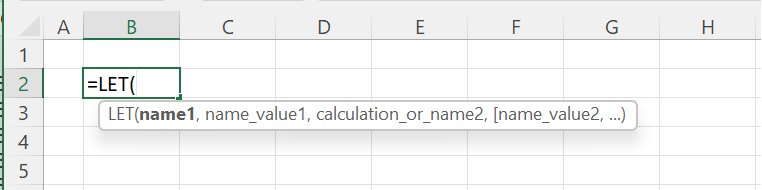### Using One Variable

A variable can refer to a numbers, text, boolean, cell ranges, other functions or formulas.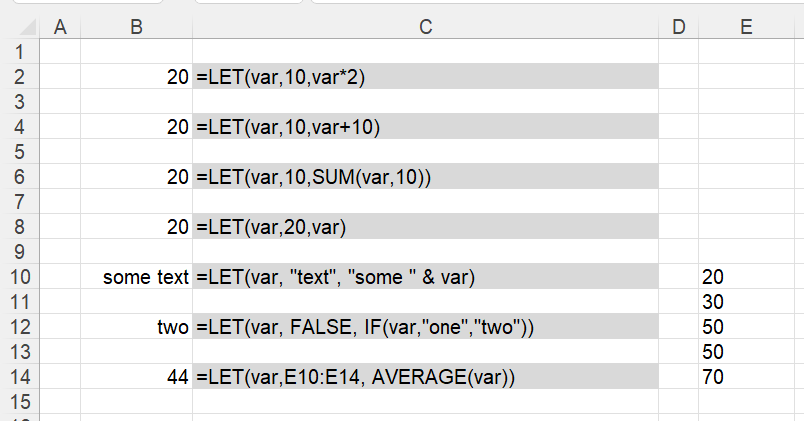### Using Two Variables

For slightly more complicated calculations and formulas you can include more than one variable.
This function can support up to 126 variables.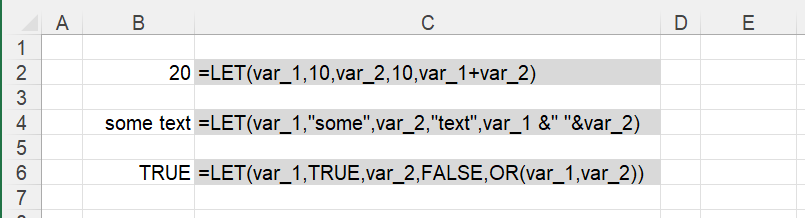### Variable Names

Variable names cannot include spaces or punctuation, although underscore can be used.
Variable names cannot be cell addresses, for example: V1, AA1, VAR1 etc.
Your naming convention should be different to the naming convention you are using for named ranges.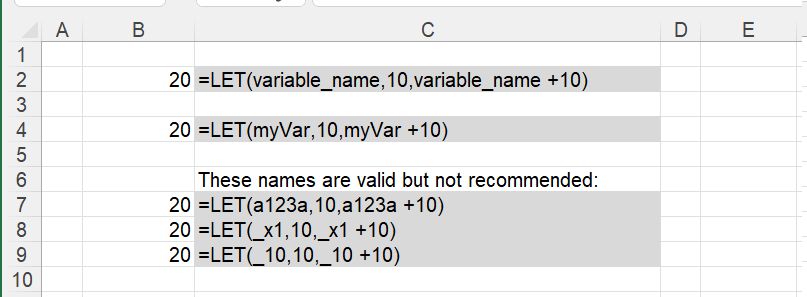If the LET calculation uses names that already exist in the Name Manager then these will be ignored.
If you misspell any of the variable names in the calculation, then #NAME? is returned.

### Nested Functions

It is possible to nest one LET function inside another LET function.
LET(myvar,"mon", LET(myvar,"true",myvar))
LET(myvar1, "mon", LET(myvar2, "tue", myvar1 & " " & myvar2))

### Dynamic Arrays

This function can be used alongside functions that return dynamic arrays.
LET(myvar, 0, OFFSET(A1:B4, myvar, 0) )

### Avoid Duplicates

This function can be used so you do not have to duplicate expressions or functions in one formula.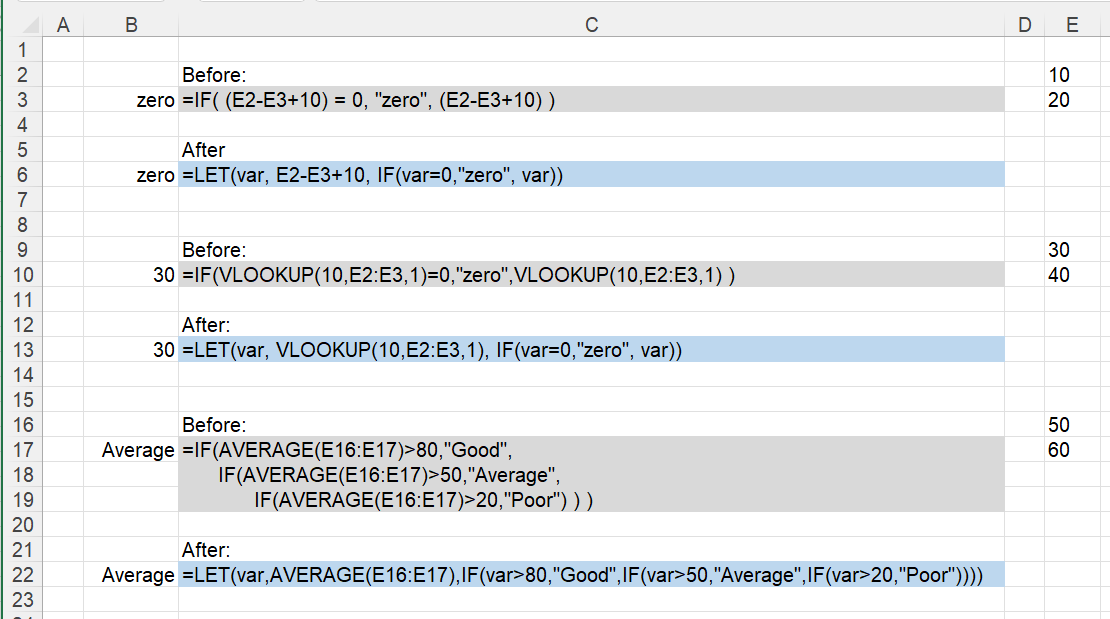### Replace Named Ranges

This could be achieved by placing the vlookup in a different cell and then using a named range.

### Faster Calculations

When the same expression is repeated in a formula multiple times Excel calculates it multiple times.
The formulas have to be fairly advanced and the data sets have to be very large (10,000+) to see a very noticeable difference in performance.